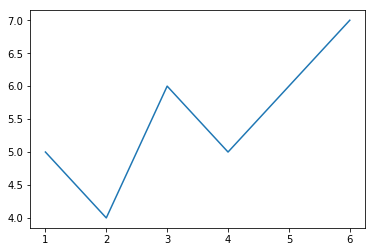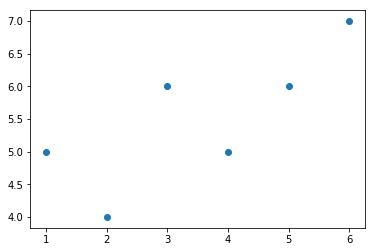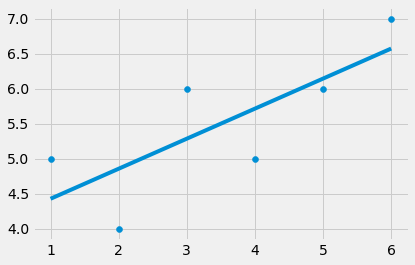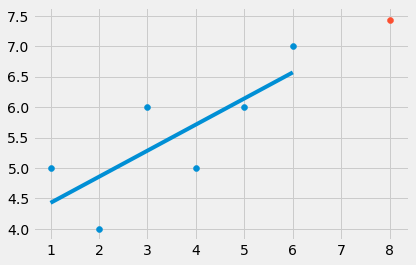• Definition:

Regression is for determining relationships between two or more variables & also used for Forcast new observation.

Exemple : X and Y are the variables.

when X = 5.(1,1), (2,2), (4,4), (100,100), (20, 20) , Y = 5 - the relationship between X and Y is Y = X

when X = 5.(1,1), (2,4), (4,16), (100,10000), (20, 400) , Y = 25 - the relationship ibetween X and Y is Y = X * X

In these two examples, we can determine the relationship between two given variables (X and Y) because we could easily identify the relationship between them. Overall, machine learning works in the same way.

the computer looks at some examples and then tries to identify “the most suitable” relationship between the sets X and Y. Using this identified relationship, it will try to predict for new examples the Y.

• X is termed as the independent variable: this is the variable that explains the other one.
• Y is termed as the dependent variable: this is the variable whose values we want to explain or forecast,and its a values depend on somthing else.

Q: to know Y we must use the equation : Y = mX + b what's m & b ?

A: m is the slope.

Equation of m , the best fit line: m = ((mean X.mean Y)- (mean XY)) / ((mean X)^2 - (mean X^2))

A: b is the Y_intercept. b = (mean Y) - m(mean X).

• linear regression using python:

```from statistics import mean #import the "mean" from statistics to calculate the m & b.
import numpy as np
import matplotlib.pyplot as plt #import Matplotlib to visualize Data.

xs = [1,2,3,4,5,6]
ys = [5,4,6,5,6,7]

plt.plot(xs,ys)
plt.show() #visualize the Data as Plot designe.
``````plt.scatter(xs,ys)
plt.show() #visualize the Data as scatter designe.``````xs = np.array([1,2,3,4,5,6], dtype=np.float64) #convert to numpy array and change the data type.
ys = np.array([5,4,6,5,6,7], dtype=np.float64)

def best_fit_slope(xs,ys): #define a function to calculate the m ( the slope of the best fit line
m = (((mean(xs) * mean(ys))- mean(xs*ys)) /
((mean(xs) *mean(xs)) - mean(xs*xs)))

return m

m = best_fit_slope(xs,ys)

print (m)
```

```0.42857142857142866
```

```def best_fit_slope(xs,ys):
m = (((mean(xs) * mean(ys))- mean(xs*ys)) /
((mean(xs) *mean(xs)) - mean(xs*xs)))
b = mean(ys) - m*mean(xs)     #calculate the intercept.
return m, b

m,b = best_fit_slope(xs,ys)

print (m,b)
```

```0.42857142857142866 4.0
```

```from matplotlib import style

style.use('fivethirtyeight')
regression_line = [(m*x)+b for x in xs] #create the line that fits the data X & Y.
plt.scatter(xs,ys)
plt.plot(xs, regression_line)
plt.show()
``````from matplotlib import style

style.use('fivethirtyeight')
regression_line = [(m*x)+b for x in xs]

predict_x = 8
predict_y = (m*predict_x)+b #Predict the value of Y.

plt.scatter(xs,ys)
plt.scatter(predict_x,predict_y)
plt.plot(xs, regression_line)
plt.show()
```